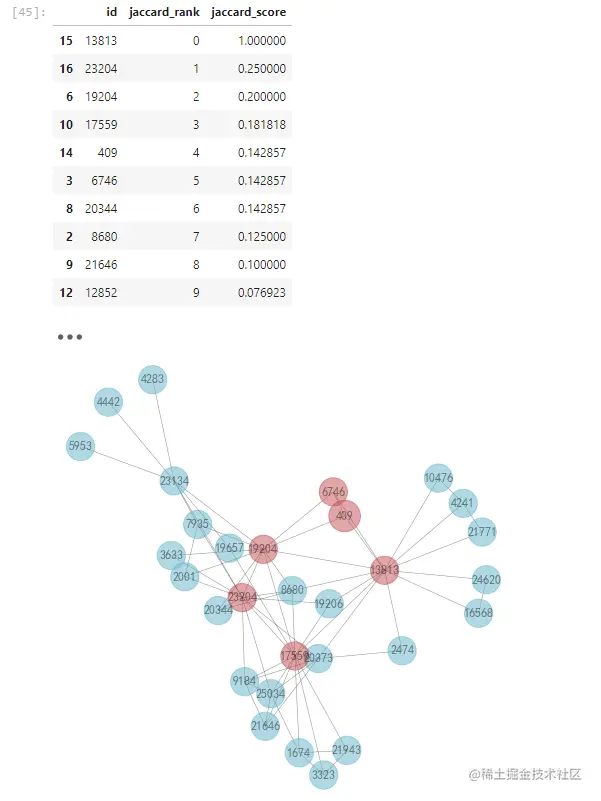# Python Networkx practice social network visualization

2022-02-01 10:24:27 ArmorHTK

「 This is my participation 11 The fourth of the yuegengwen challenge 25 God , Check out the activity details ：2021 One last more challenge

Use python-networkx Practice social networking visualization

# 01 Use networkx Make map network model

• Use networkx Create diagrams , Add nodes and edges
• Look at the adjacency matrix / Adjacency list / Fig. thermodynamic diagram
• Figure network weighting
• Figure network statistics （ Out and in 、 Shortest path ）

Guide pack

import networkx as nx
import matplotlib.pyplot as plt
import collections
import random
import numpy as np
from pylab import rcParams
import warnings
warnings.filterwarnings("ignore")
#  Support Chinese
plt.rcParams['font.sans-serif'] = ['SimHei']  #  Used to display Chinese labels normally
plt.rcParams['axes.unicode_minus'] = False  #  Used to display negative sign normally
Copy code 

## Add a node

#  Add node
G = nx.Graph() #  Create an empty undirected graph   Create a directed graph using nx.DiGraph()
G.add_node(1) #  Create a single node
G.add_nodes_from(range(10)) #  Create a set of nodes
nx.draw(G,node_color="darkblue")
Copy code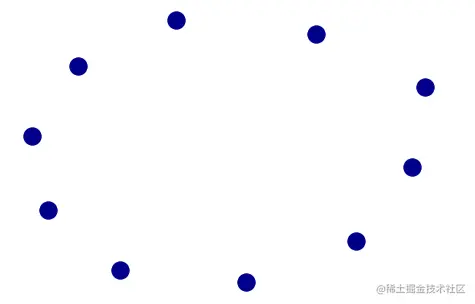G.add_edge(1,2)
e = [(2,3),(9,3),(8,4),(3,5),(8,6),(0,8),(1,9)]
nx.draw(G,with_labels=True,node_color="darkblue",font_color="white")
Copy code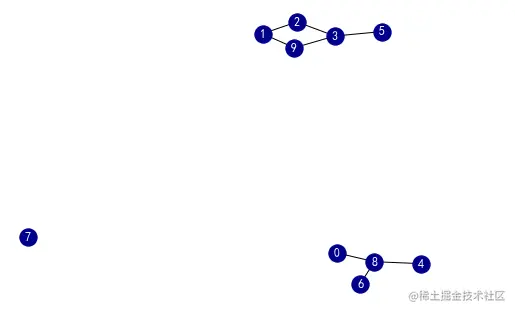## Print adjacency matrix and adjacency table

#  Print adjacency matrix
print(" Adjacency matrix ")
print("="*50)
print(" Adjacency list ")
for i in G.adj.items():
print(i)
Copy code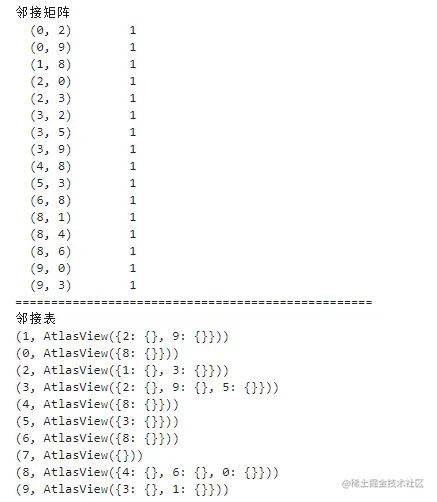## Heat map

#  Thermodynamic diagram of adjacency matrix ： Response sparsity
print(nx.to_numpy_matrix(G)) # Convert adjacency matrix to numpy Format
plt.imshow(nx.to_numpy_matrix(G)) # establish 2 Dimensional thermodynamic diagram
cbar = plt.colorbar() #-->  Set up colorbar Thermal diagram caption
cbar.set_ticks([0,1]) #-->  Set up colorbar Scale range
cbar.ax.set_yticklabels(['Zero','One'],) #-- Set up colorbar The name of the head and tail coordinates of the scale axis
cbar.set_label('link', rotation=0) #-- Set up colorbar Coordinate name
plt.xlabel('node idx') #-- Set up  x  Axis
plt.ylabel('node idx') #-- Set up  y  Axis
Copy code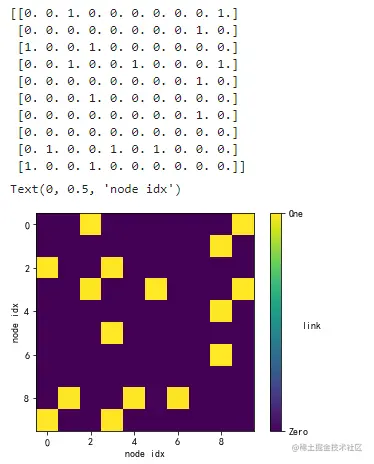## Empowerment

#  Empowerment
for e in G.edges():
G[e][e]['weight'] = random.uniform(0, 1) #  Use uniform Distribute and re empower existing relationships
print(nx.to_numpy_matrix(G))
plt.imshow(nx.to_numpy_matrix(G))
cbar = plt.colorbar() #-->  Set up colorbar Thermal diagram caption
cbar.set_ticks([0,1]) #-->  Set up colorbar Scale range
cbar.ax.set_yticklabels(['Zero','One'],) #-- Set up colorbar The name of the head and tail coordinates of the scale axis
cbar.set_label('link', rotation=0) #-- Set up colorbar Coordinate name
plt.xlabel('node idx') #-- Set up  x  Axis
plt.ylabel('node idx') #-- Set up  y  Axis
Copy code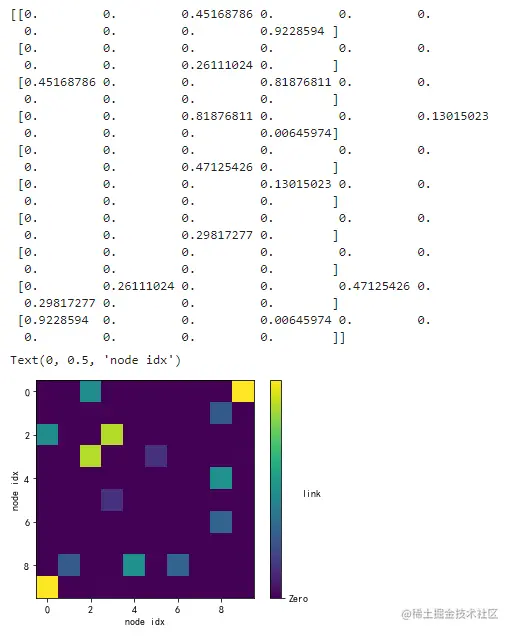## Network statistics

#####  Network statistics
#  degree
G =nx.karate_club_graph() #  Create a karate membership club chart  ： This is a very famous social network diagram
degree_sequence = sorted([d for n, d in G.degree()], reverse=True) #  Save the degree of each node , And in descending order
print(" degree   array ", degree_sequence)
print("= "*50)
degreeCount = collections.Counter(degree_sequence) #  Calculate the number of degrees for each
print(" degree   frequency ", degreeCount)
print("= "*50)

deg, cnt = zip(*degreeCount.items()) #  Create an iterator
rcParams['figure.figsize'] = 12, 8 #  Set the global size of the sketchpad
fig, ax = plt.subplots() #  Create subgraphs

plt.bar(deg, cnt, width=0.80, color='darkblue')
plt.title(" degree   Histogram ")
plt.ylabel(" frequency ")
plt.xlabel(" degree ")
plt.axes([0.4, 0.4, 0.5, 0.5])
plt.axis('off')
ax.set_xticks([d + 0.4 for d in deg])
ax.set_xticklabels(deg)
pos = nx.spring_layout(G) #--  Set up the network layout
nx.draw_networkx_nodes(G, pos, node_color= 'darkblue',node_size=20) #  drawing
nx.draw_networkx_edges(G, pos, alpha=0.5) #  Draw edge
plt.show()
print(' The degree mean of an undirected graph ：', np.mean(G.degree()))
Copy code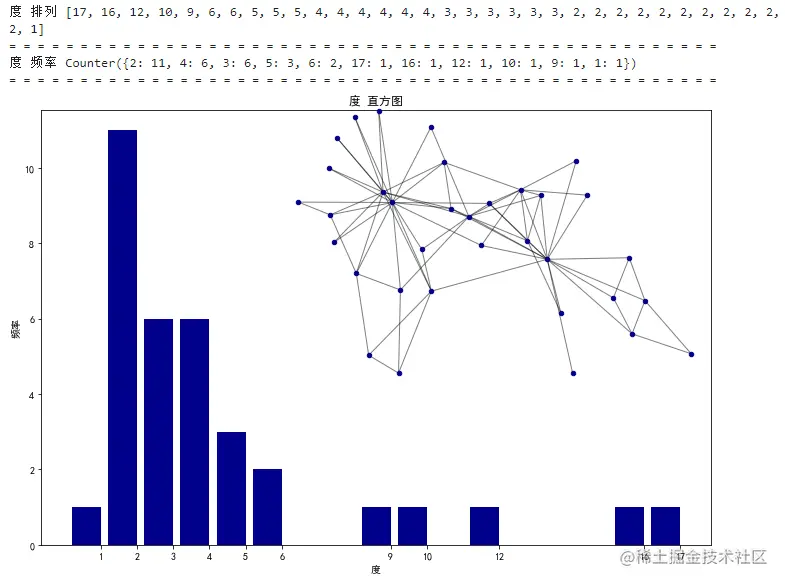The degree mean of an undirected graph ： 10.544117647058824

## Shortest path

#  Shortest path
R = nx.karate_club_graph()
source=14 #  Choose a point as the starting point
target=16 #  Choose a point to finish

#  Shortest path mapping function
def short_path_plot(G,source,target):
pos = nx.spring_layout(G) #--  Set up the network layout
nx.draw(G,pos,node_color='k', with_labels=True, font_color='white') #--  Drawing structure

path = nx.shortest_path(G,source=14,target=16) #--  call shortest path Calculate the shortest path
print(" Shortest path ：",path)

path_edges = list(zip(path,path[1:])) #--  Create shortest path edge
nx.draw_networkx_nodes(G,pos,nodelist=path,node_color='r', label=True)  #--  Draw node
nx.draw_networkx_edges(G,pos,edgelist=path_edges,edge_color='r',width=10)  #-- Draw edge
plt.axis('equal')
plt.show()
return

# Call function
short_path_plot(R,source,target)
Copy code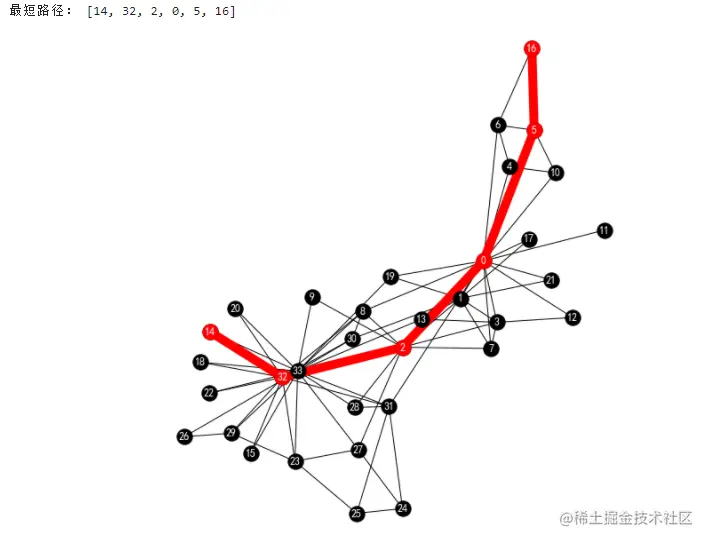# 02 Network structure

• Random graphs
• Small world model
• Scale free network
#  download   Power law distribution   package
# !pip install powerlaw

import re
import powerlaw
import seaborn as sns
from operator import itemgetter

#  Histogram drawing
def draw_hist_network(G):
degree_sequence = sorted([d for n, d in G.degree()], reverse=True)
degreeCount = collections.Counter(degree_sequence)
deg, cnt = zip(*degreeCount.items())
rcParams['figure.figsize'] = 10, 5
fig, ax = plt.subplots()
plt.bar(deg, cnt, width=0.80, color='darkblue')
plt.title(" degree   Histogram ")
plt.ylabel(" frequency ")
plt.xlabel(" degree ")
ax.set_xticks([d + 0.4 for d in deg])
ax.set_xticklabels(deg)
plt.axes([0.4, 0.4, 0.5, 0.5])
Gcc = G.subgraph(sorted(nx.connected_components(G), key=len, reverse=True))
pos = nx.spring_layout(G)
plt.axis('off')
nx.draw_networkx_nodes(G, pos, node_color= 'darkblue',node_size=20)
nx.draw_networkx_edges(G, pos, alpha=0.4)
plt.show()
pass
#  Network drawing
def draw_network(G,name):
plt.title(name)
pos = nx.circular_layout(G)
nx.draw_networkx_nodes(G, pos, node_color= 'darkblue',node_size=20)
nx.draw_networkx_edges(G, pos, alpha=0.4)
plt.axis('off')
plt.show()
pass
Copy code 

## Random graphs

#  Generate random graph
nodes_n=50
degree=20
simulation_number=10
rcParams['figure.figsize'] = 5, 5

# Connectivity  = 0.01:
#------------------------------
G = nx.random_regular_graph(degree,nodes_n) #<-- Generate the Small World Network
draw_network(G,name=" Random positive Taiyuan distribution ")
Copy code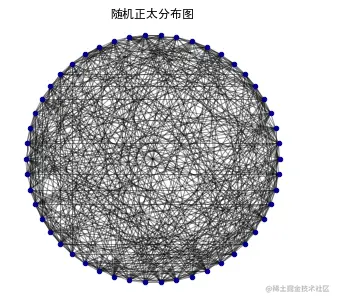## Small world network

#  Small world network
nodes_n=1000
degree=20
rcParams['figure.figsize'] = 5, 5

# Simple small world model ------------------------------
G = nx.watts_strogatz_graph(20,5,p=0.01) #<--  Generate a small world model
draw_network(G,name=" Small world model ")
# Connectivity  = 0.01:
#------------------------------
G = nx.watts_strogatz_graph(nodes_n,degree, p = 0.01) #<--  Generate a small world model
draw_hist_network(G)

# Connectivity  = 0.05:
#------------------------------
G = nx.watts_strogatz_graph(nodes_n,degree, p = 0.05) #<--  Generate a small world model
draw_hist_network(G)

# Connectivity  = 0.1:
#------------------------------
G = nx.watts_strogatz_graph(nodes_n,degree, p = 0.1) #<--  Generate a small world model
draw_hist_network(G)
Copy code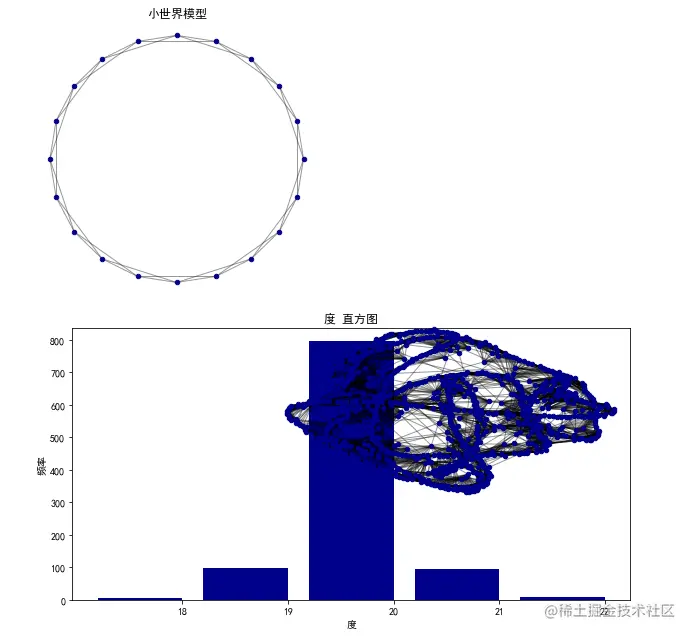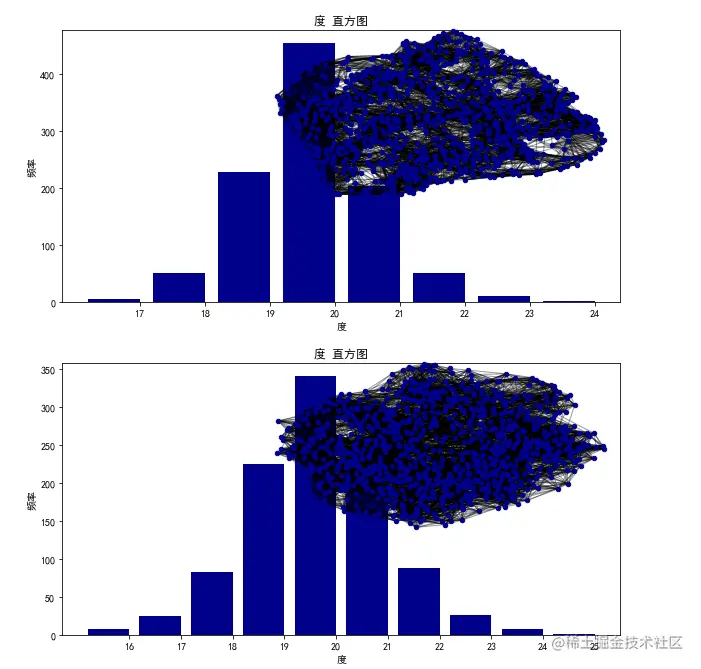## Scale free network

The scale-free network presents a power-law distribution

#  Scale free network
node_n = 100000 #--  Number of nodes
m=3   #--  The number of edges attached from the new node to the existing node
G = nx.barabasi_albert_graph(node_n, m)
# draw_network(G,name=" Scale free network ") # 100000 Node drawing is very slow and takes up memory
degree_freq = nx.degree_histogram(G)
degrees = range(len(degree_freq))
plt.figure(figsize=(12, 8))
plt.loglog(degrees[m:], degree_freq[m:],'go-')
plt.xlabel(' degree ', fontsize = 'x-large')
plt.ylabel(' frequency ', fontsize = 'x-large')
plt.show()
Copy code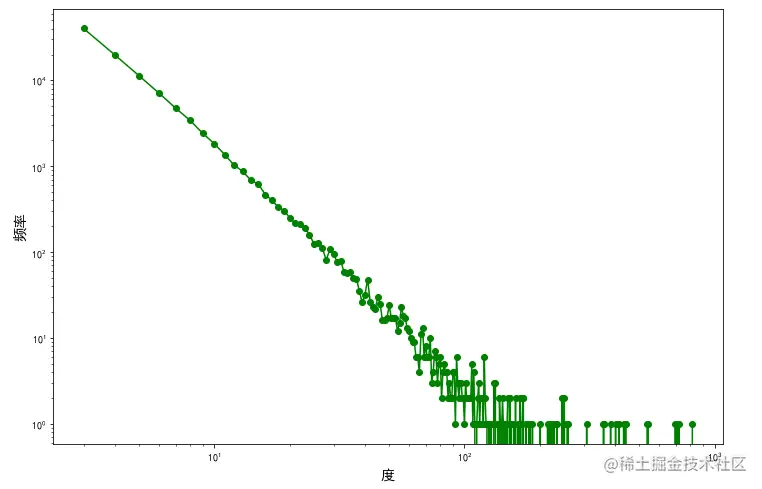# 03 Node measurement - Centrality

## What is centrality ？

Centrality is a term that describes the importance of each node in a diagram . In order to answer “ That node is the most important node in the chart （ The vertices ）？” This is a problem , A large number of experiments have been carried out , The list of experiments is as follows ：

• Point degree, center degree Degree Centrality
• Intermediary centrality Betweenness Centrality
• Near centrality Closeness Centrality
• Centrality of eigenvector Eigenvector Centrality
• Katz centrality Katz Centrality

Point degree, center degree / Intermediary centrality / Absolute centrality is used when approaching centrality

import pandas as pd
import matplotlib.colors as mcolors
from pylab import rcParams
Copy code 

Zachary Karate Club is a widely used social network , The nodes represent members of the Karate Club , Edges represent the relationship between members .

# Zachary  Karate Club
G = nx.karate_club_graph()
pos = nx.circular_layout(G) #--  Disc layout
nodes = nx.draw_networkx_nodes(G, pos, node_size=400,node_color='White',edgecolors='b')
nodes.set_norm(mcolors.SymLogNorm(linthresh=0.03, linscale=2))
labels = {i:i for i in G.nodes()}
labels = nx.draw_networkx_labels(G, pos, labels, font_size=12)
edges = nx.draw_networkx_edges(G, pos, edge_color = 'grey')
plt.axis('off')
plt.show()

def draw(G, pos, measures, measure_name):
nodes = nx.draw_networkx_nodes(G, pos, node_size=400, cmap=plt.cm.plasma,
node_color=list(measures.values()),
nodelist=list(measures.keys()))
nodes.set_norm(mcolors.SymLogNorm(linthresh=0.03, linscale=1))
labels = nx.draw_networkx_labels(G, pos, font_color='white')
edges = nx.draw_networkx_edges(G, pos)
plt.title(measure_name,size=18)
cbar = plt.colorbar(nodes)
cbar.set_label(' Centrality weight ', rotation=0)
plt.axis('off')
plt.show()
pass
Copy code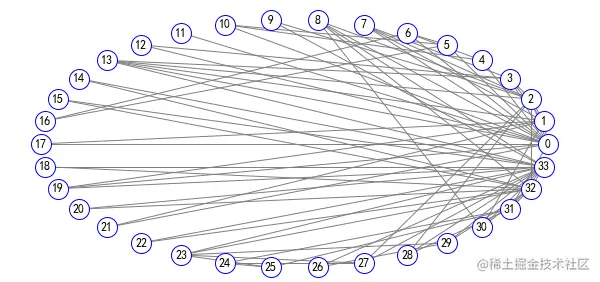## Point degree, center degree

#  Point degree, center degree  Degree Centrality
draw(G, pos,nx.degree_centrality(G),' Point degree, center degree ')
Copy code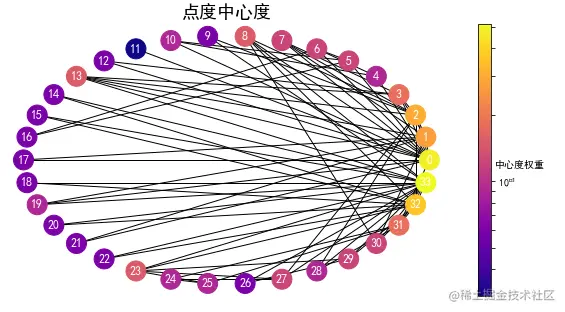## Intermediary centrality

#  Intermediary centrality  Betweenness Centrality
draw(G, pos, nx.betweenness_centrality(G), ' Intermediary centrality ')
Copy code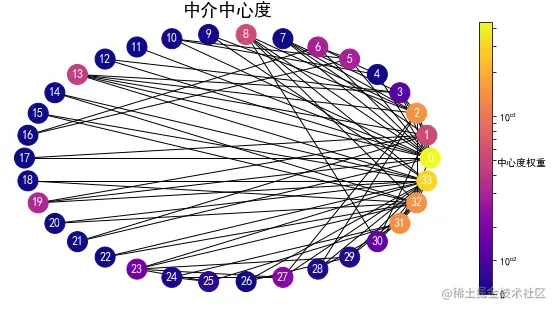## Near centrality

#  Near centrality  Closeness Centrality
draw(G, pos, nx.closeness_centrality(G), ' Near centrality ')
Copy code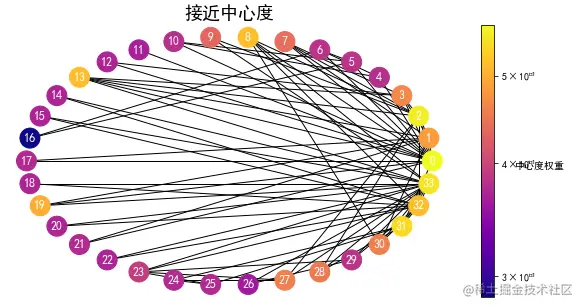## Centrality of eigenvector

#  Centrality of eigenvector  Eigenvector Centrality
draw(G, pos, nx.eigenvector_centrality(G), ' Centrality of eigenvector ')
Copy code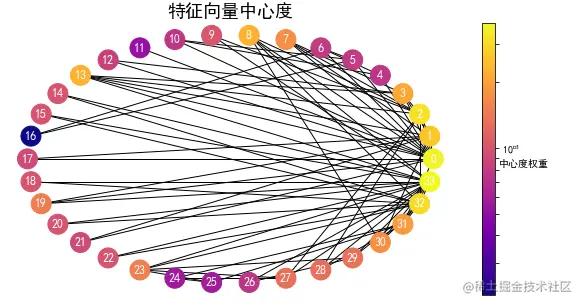## Katz centrality

#  Katz centrality  Katz Centrality
draw(G, pos, nx.katz_centrality(G, alpha=0.1, beta=1.0), ' Katz centrality ')
Copy code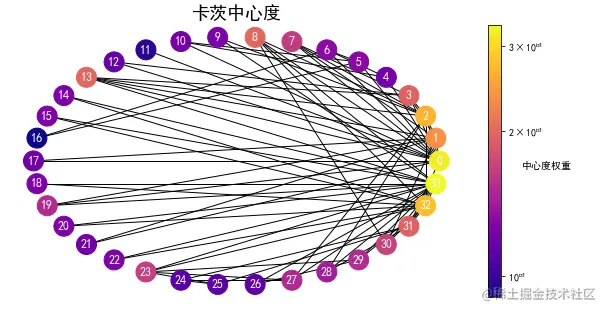# Create a pandas Table compares various centrality :
dataset1=[]
for k in nx.degree_centrality(G).keys():
dataset1.append([k,nx.degree_centrality(G)[k],nx.katz_centrality(G)[k],nx.eigenvector_centrality(G)[k],
nx.closeness_centrality(G)[k],nx.betweenness_centrality(G)[k]])
rcParams['figure.figsize'] = 5, 5
sns.set(style="ticks")
# establish dataframe:
df=pd.DataFrame(dataset1,columns=['id',
'Degree centrality','Katz centrality','Eigenvector centrality',
'Clossness centrality','Betweenness centrality'])
# Output   The relationship between two variables :
sns.pairplot(df)
Copy code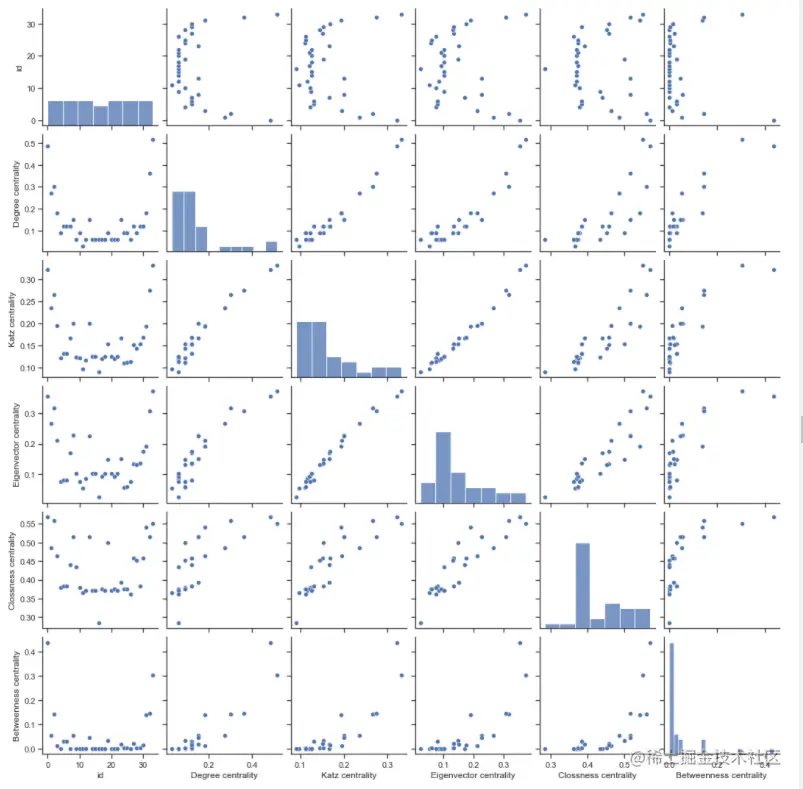# 04. Overall measurement

• density
• Central potential
#  density
G = nx.karate_club_graph()
print('karate_club_graph chart   density ：', nx.density(G))

G = nx.watts_strogatz_graph(21,7,p=0.05) #<--  Generate a small world model
print(' The generated small world model   density ：', nx.density(G))
Copy code 

karate_club_graph chart density ： 0.13903743315508021 The generated small world model density ： 0.3

## density

1. density
The density of an undirected graph is $D=\frac{2m}{n(n-1)}$ The density of a digraph is $D=\frac{m}{n(n-1)}$ among n yes G Number of nodes in m yes G The number of middle edges .
The density of an unbounded graph is 0, The density of the complete graph is 1, The density of a multigraph can be greater than 1.

## Central potential

2. Central potential : The central potential is used to measure the overall centrality of the network

1. First, find the maximum centrality value in the network MAX;
2. Then calculate the... Separately MAX The difference between the value and the centrality of other points “ Difference value ”;
3. Then calculate these " Difference value ” The sum of ;
4. Finally, divide this sum by the theoretical maximum possible value of the sum of each difference .

The absolute central potential is $C_{AD}= \frac{\sum_{i=1}^{n}(C_{ADmax}-C_{ADi})}{(n-1)(n-2)}$

The relative central potential is $C_{RD}= \frac{\sum_{i=1}^{n}(C_{RDmax}-C_{RDi})}{(n-2)}$

#  Point degree central potential
n = nx.number_of_nodes(G) #-- n Number of nodes
centrality_list = np.array(list(nx.degree_centrality(G).values())) #  Centrality list
max_centrality = max(centrality_list) #  Centrality   Maximum
degree_centralization = sum(max_centrality - centrality_list) / (n-1)*(n-2)
if mode=='RD':
degree_centralization = sum(max_centrality - centrality_list) / (n-2)
return degree_centralization
Copy code 
G = nx.karate_club_graph()
print('karate_club_graph chart   Absolute central potential ：', Degree_Centralization(G,mode='AD'))
print('karate_club_graph chart   Relative central potential ：', Degree_Centralization(G,mode='RD'))
draw_network(G,name="")

G = nx.watts_strogatz_graph(21,7,p=0.05) #<--  Generate a small world model
print(' The generated small world model   Absolute central potential ：', Degree_Centralization(G,mode='AD'))
print(' The generated small world model   Relative central potential ：', Degree_Centralization(G,mode='RD'))
draw_network(G,name="")
Copy code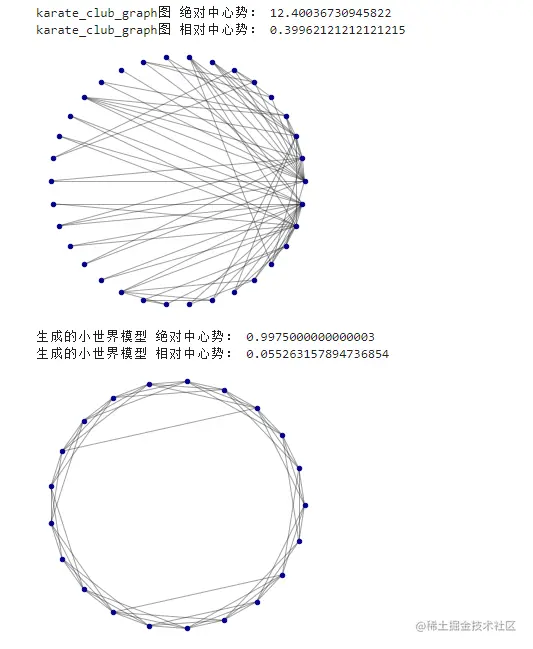# 05. Agglomerative subgroup

• K- nucleus Agglomerative subgroup
G = nx.karate_club_graph()
nx.core_number(G)

#  Output
{0: 4,
1: 4,
2: 4,
3: 4,
4: 3,
5: 3,
6: 3,
7: 4,
8: 4,
9: 2,
10: 3,
11: 1,
12: 2,
13: 4,
14: 2,
15: 2,
16: 2,
17: 2,
18: 2,
19: 3,
20: 2,
21: 2,
22: 2,
23: 3,
24: 3,
25: 3,
26: 2,
27: 3,
28: 3,
29: 3,
30: 4,
31: 3,
32: 4,
33: 4}
Copy code 
def draw_clu(G, pos, measures, measure_name):
clusters=np.array(list(set(measures.values())))
plt.figure()
nodes = nx.draw_networkx_nodes(G, pos, node_size=250, cmap=mcolors.ListedColormap(plt.cm.Set3(clusters)),
node_color=np.array(list(measures.values()))-1,
nodelist=list(measures.keys()))
print(measures.values())
print(measures.keys())
labels = nx.draw_networkx_labels(G, pos)
edges = nx.draw_networkx_edges(G, pos)
plt.title(measure_name)
rcParams['figure.figsize'] = 12, 8
rcParams['font.sans-serif'] = ['SimHei']
cb = plt.colorbar(nodes,ticks=range(0,len(clusters)), label=' Subgroup label ')
cb.ax.tick_params(length=0)
cb.set_ticklabels(list(set(measures.values())))
nodes.set_clim(-0.5, len(clusters)-0.5)
plt.axis('off')
plt.show()

G = nx.karate_club_graph()
pos = nx.spring_layout(G)
draw_clu(G, pos, nx.core_number(G),'k-Core')
Copy code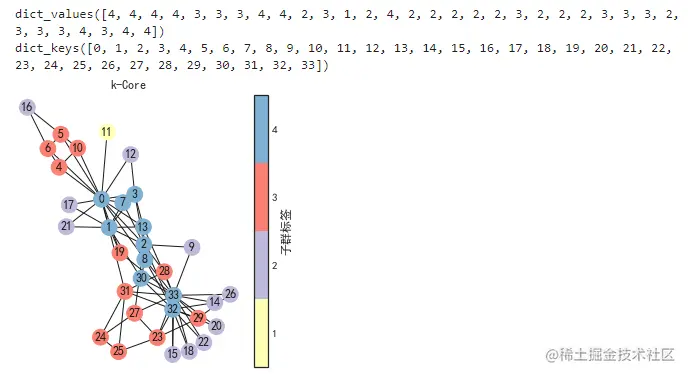draw(G, pos, nx.core_number(G), 'k-Core')
Copy code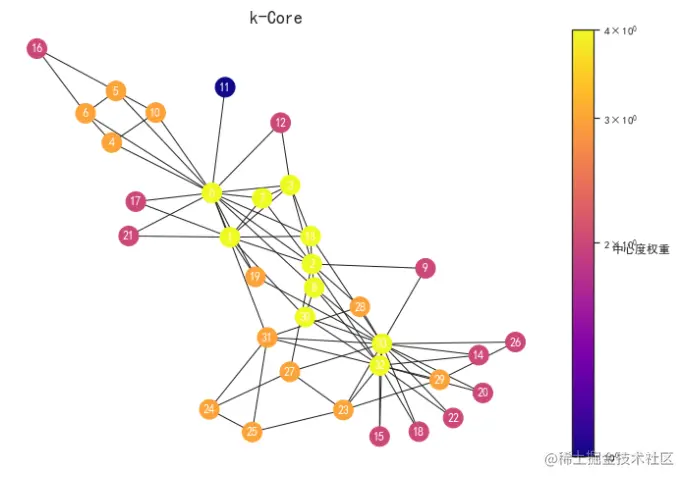# 06. Link prediction

import networkx as nx
import numpy as np
import urllib.request
urllib.request.urlretrieve("http://snap.stanford.edu/data/ca-GrQc.txt.gz", "ca-GrQc.txt.gz")
graph.order()
draw_network(graph,name="")
Copy code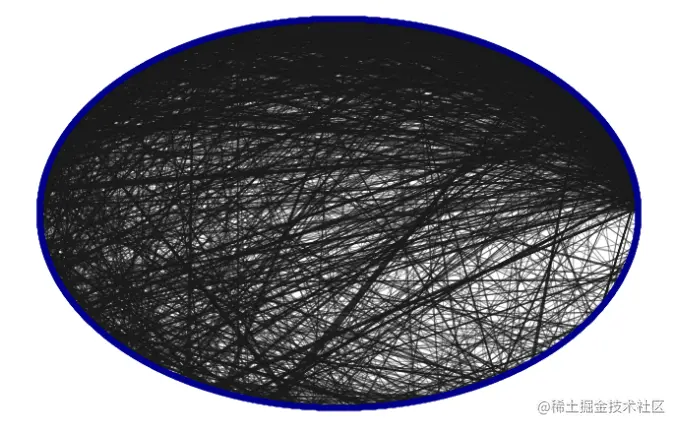degrees = dict(graph.degree())
author = sorted(degrees.items(),key=lambda x: x,reverse=True)
print(' scholars  %s  Of " degree " by  %d' % (author, graph.degree()[author]))

#  Get subgraph
def get_subgraph(graph, nodes, n=100):
neighbors = set()
for ni in nodes:
neighbors |= set(graph.neighbors(ni))
# plot at least the target node and his neighbors.
result = set(nodes) | neighbors
# add "friends of friends" up to n total nodes.
for x in neighbors:
# how many more nodes can we add?
maxsize = n - len(result)
toadd = set(graph.neighbors(x)) - result
if len(result) > n:
break
return graph.subgraph(result)

subgraph = get_subgraph(graph, [author], n=30)
print(' The subgraph has a  %d  node ' % len(subgraph.nodes()))
Copy code 

scholars 13813 Of " degree " by 13
The subgraph has a 30 node

#  Draw a subgraph
def plot_subgraph(subgraph, target_nodes):
nodes = list(subgraph.nodes())
colors = ['c'] * len(nodes)
for n in target_nodes:
idx = nodes.index(n)
colors[idx] = 'r'
sizes =  * len(nodes)
sizes[idx] = 1000
plt.figure(figsize=(8,8))
plt.axis('off')
nx.draw_networkx(subgraph, nodelist=nodes, with_labels=True,
width=.5, node_color=colors,
node_size=sizes, alpha=.5)

plot_subgraph(subgraph, [author])
Copy code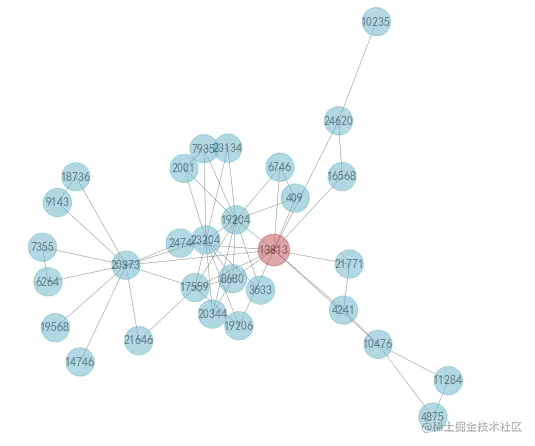The author should be recommended 13813 With whom ？

## Connection prediction method

• The link prediction task is regarded as the ranking problem in information retrieval
• Calculate the new fraction for each edge $s(X,Y)$.
• Arrange all possible fractional edges in descending order $s(X,Y)$.
• Select the highest fractional edge $s(X,Y)$ $\rightarrow$.

1.) Shortest path ： $s(X,Y) =$ from $X$ To $Y$ The length of the shortest path .

#  Shortest path algorithm
def rank_by_shortest_path(graph, node):
paths = nx.shortest_path_length(graph, node)
return sorted(paths.items(), key=lambda x: x)

shortest_paths = rank_by_shortest_path(graph, author)
print(' Shortest path  top-20 :')
shortest_paths[:20]
print(" By author 13813 As the starting point , The shortest path in the network is 2 Our partners are %s position " % len([s for s in shortest_paths if s == 2]))
print(' Link prediction  top-10 :')
[s for s in shortest_paths if s == 2][:10]
Copy code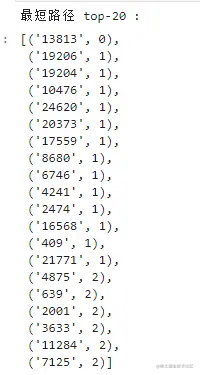By author 13813 As the starting point , The shortest path in the network is 2 Our partners are 57 position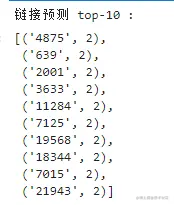visualization , Recommendation effect

pair = set([author, '5227','5543','10931','639','18736'])
plot_subgraph(get_subgraph(graph, pair, n=30), pair)

Copy code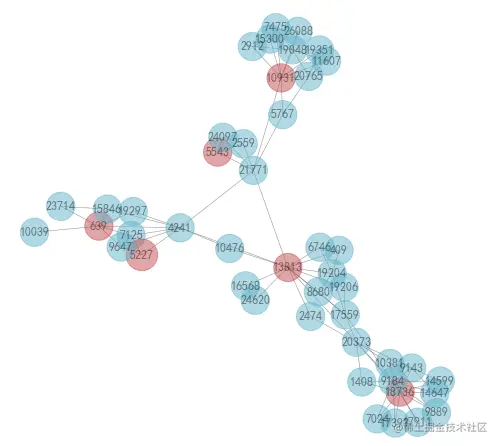Another way ： If $X$ and $y$ There are many co authors , that $x$ and $y$ More likely to co-author

2.) jaccard： $S = \frac{|N(X) \cap N(Y)|}{|N(X) \cup N(Y)|}$

def rank_by_jaccard(graph, node):
neighbors = set(graph.neighbors(node))
scores = []
for n in graph.nodes():
neighbors2 = set(graph.neighbors(n))
scores.append((n, len(neighbors & neighbors2) / len(neighbors | neighbors2)))
return sorted(scores, key=lambda x: x, reverse=True)

common_jaccard = rank_by_jaccard(graph, author)
common_jaccard[:20]
plt.plot(sorted([x for x in common_jaccard if x > 0.]))
plt.show()
Copy code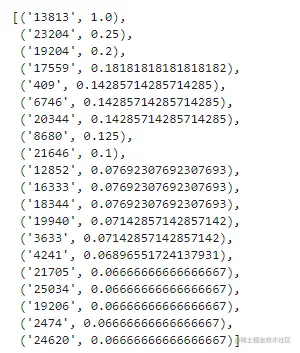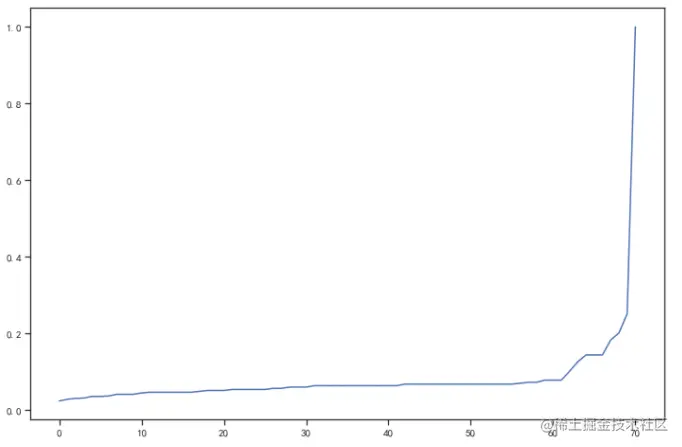def dataframe_scores(scores_list, names, n):
ids = set()
for scores in scores_list:
ids.update([x for x in scores[:n]])
print('jaccard Algorithm link prediction  top %d' % (n))
results = []
for id_ in ids:
result = {'id': id_}
for scores, name in zip(scores_list, names):
for rank, (id2, score) in enumerate(scores):
if id2 == id_:
result[name + '_rank'] = rank
result[name + '_score'] = score
break
results.append(result)
for name in names:

df = dataframe_scores([common_jaccard],['jaccard'],20)

pair = set([author, '23204','19204','17559','409','6746'])
plot_subgraph(get_subgraph(graph, pair, n=30), pair)
Copy code